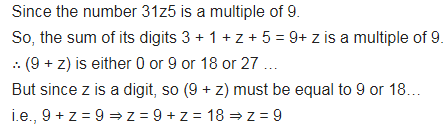# If 31z5 is a multiple of 9, where z is a digit, what is the value of z?

If 31z5 is a multiple of 9, where z is a digit, what is the value of z?